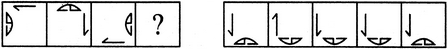# Non Verbal Reasoning - Analogy - Discussion

Discussion Forum : Analogy - Section 1 (Q.No. 18)
Directions to Solve

Each of the following questions consists of two sets of figures. Figures A, B, C and D constitute the Problem Set while figures 1, 2, 3, 4 and 5 constitute the Answer Set. There is a definite relationship between figures A and B. Establish a similar relationship between figures C and D by selecting a suitable figure from the Answer Set that would replace the question mark (?) in fig. (D).

18.

Select a suitable figure from the Answer Figures that would replace the question mark (?).(A)     (B)      (C)     (D)                  (1)      (2)      (3)      (4)      (5)
1
2
3
4
5
Explanation:
Both the elements move to the adjacent side in a CW direction; the half-arrow rotates 90o ACW; the semi-circular element rotates 90oACW and gets laterally inverted.
Discussion:
4 comments Page 1 of 1.

Adeeba yousuf said:   1 year ago
@All.

Look there is a relation between A and C so we should establish a relation between B and D. A and C are opposite to each other for the line but the half-circle will also be opposite.

Khemu Singh said:   1 year ago
How it will be D? Please explain it clearly.

Subu said:   8 years ago
How's it possible? Can any one tell me?